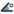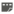# Difference between revisions of "Dev:Types"

### ValueNode Types

Each ValueNode (and hence each Parameter) in Synfig has one of 13 types:

1.Bool -- a boolean value; true or false. Represented in Studio as a checkbox
2.Integer -- an integer value; a whole number. -3 or 0 or 2 are examples.
3.Real -- a [http://en.wikipedia.org/wiki/Real_number real number}}. -3.0, 0.0, 2.0 and 3.1415 are examples.
4.Angle -- a real number that is interpreted as an angle
5.Time -- a time, displayed in days, hours, minutes, seconds and frames
6.Vector -- a 2d vector. usually represented as two Reals representing X and Y values
7.Color -- a color. Usually represented as Red/Green/Blue/Alpha values
8.Segment -- a single bezier curve segment. Defined by 4 Vectors, representing 2 Vertices and 2 Tangents
9.SplinePoint -- a point in a Spline. Defined by 6 parameters (a Vector defining its location, a Real defining its width, another Real defining its origin, a Bool defining whether its tangents are split or not, and two Vectors defining the direction of its tangents (only the first of which is used if the tangents aren't split)
10.List -- A list. For example, an outline's Vertices parameter is simply a list of SplinePoint valuenodes.
11.Canvas -- A collection of layers. When you group layers, you're creating a new Group Layer called, by default "Group". The group layer has a parameter called 'Canvas' with type 'Canvas' and its value is the group of layers you've grouped.
12.String -- a string of characters, for example "Hello Mum!"
13.Gradient -- a range of colors (a mapping from a real parameter to a color). Can be defined in many ways, but is usually editing using the gradient editor.